Vectors - Motion and Forces in Two Dimensions - Lesson 3 - Forces in Two Dimensions

# Resolution of ForcesEarlier in Lesson 1, the method of resolving a vector into its components was thoroughly discussed. During that lesson, it was said that any vector that is directed at an angle to the customary coordinate axis can be considered to have two parts - each part being directed along one of the axes - either horizontally or vertically. The parts of the single vector are called components and describe the influence of that single vector in that given direction. One example that was given during Lesson 1 was the example of Fido being pulled upon by a dog chain. If the chain is pulled upwards and to the right, then there is a tensional force acting upwards and rightwards upon Fido. That single force can be resolved into two components - one directed upwards and the other directed rightwards. Each component describes the influence of that chain in the given direction. The vertical component describes the upward influence of the force upon Fido and the horizontal component describes the rightward influence of the force upon Fido.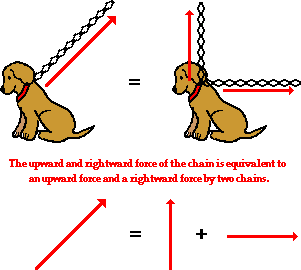### Determining the Components of a Vector

The task of determining the amount of influence of a single vector in a given direction involves the use of trigonometric functions. The use of these functions to determine the components of a single vector was also discussed in Lesson 1 of this unit. As a quick review, let's consider the use of SOH CAH TOA to determine the components of force acting upon Fido. Assume that the chain is exerting a 60 N force upon Fido at an angle of 40 degrees above the horizontal. A quick sketch of the situation reveals that to determine the vertical component of force, the sine function can be used and to determine the horizontal component of force, the cosine function can be used. The solution to this problem is shown below.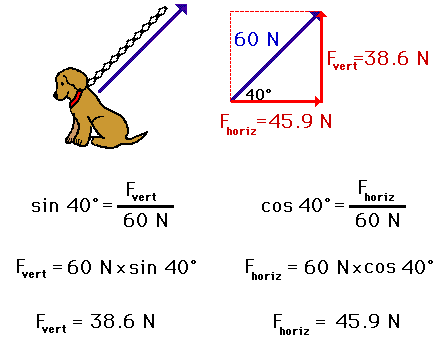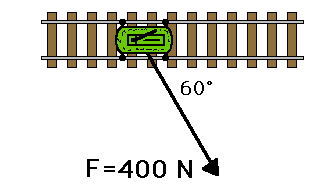As another example of the use of SOH CAH TOA to resolve a single vector into its two components, consider the diagram at the right. A 400-N force is exerted at a 60-degree angle (a direction of 300 degrees) to move a railroad car eastward along a railroad track. A top view of the situation is depicted in the diagram. The force applied to the car has both a vertical (southward) and a horizontal component (eastward). To determine the magnitudes of these two components, the sine and cosine function will have to be used. The task is made clearer by beginning with a diagram of the situation with a labeled angle and a labeled hypotenuse. Once a triangle is constructed, it becomes obvious that the sine function will have to be used to determine the vertical (southward) component and the cosine function will have to be used to determine the horizontal (eastward) component. The triangle and accompanying work is shown below.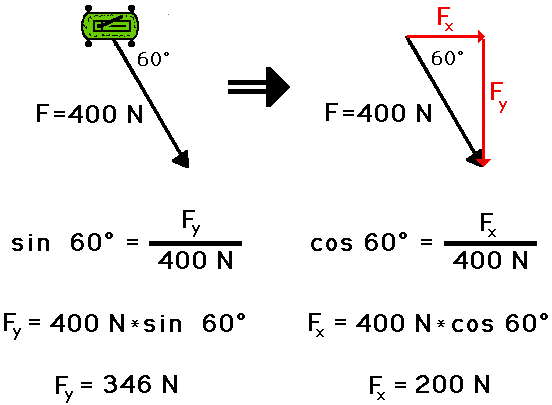Anytime a force vector is directed at an angle to the horizontal, the trigonometric functions can be used to determine the components of that force vector. To assure that you understand the use of SOH CAH TOA to determine the components of a vector, try the following three practice problems. To view the answers, click on the button.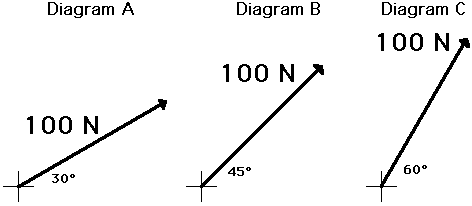An important concept is revealed by the above three diagrams. Observe that the force is the same magnitude in each diagram; only the angle with the horizontal is changing. As the angle that a force makes with the horizontal increases, the component of force in the horizontal direction (Fx) decreases. The principle makes some sense; the more that a force is directed upwards (the angle with the horizontal increases), the less that the force is able to exert an influence in the horizontal direction. If you wish to drag Fido horizontally, then you would make an effort to pull in as close to a horizontal direction as possible; you would not pull vertically on Fido's chain if you wish to pull him horizontally.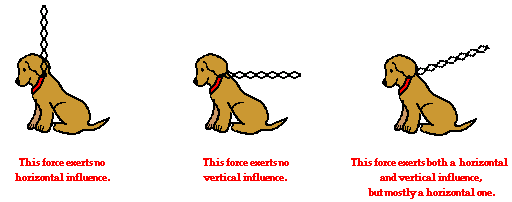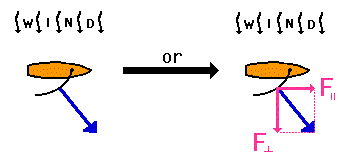One important application of this principle is in the recreational sport of sail boating. Sailboats encounter a force of wind resistance due to the impact of the moving air molecules against the sail. This force of wind resistance is directed perpendicular to the face of the sail, and as such is often directed at an angle to the direction of the sailboat's motion. The actual direction of this force is dependent upon the orientation of the sail. To determine the influence of the wind resistance force in the direction of motion, that force will have to be resolved into two components - one in the direction that the sailboat is moving and the other in a direction perpendicular to the sailboat's motion. See diagram at right. In the diagram below, three different sail orientations are shown. Assuming that the wind resistance force is the same in each case, which case would produce the greatest influence in the direction of the sailboat's motion? That is, which case has the greatest component of force in the direction parallel to the boats' heading?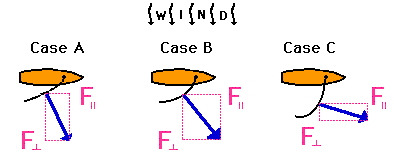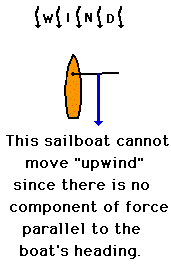Many people believe that a sailboat cannot travel "upwind." It is their perception that if the wind blows from north to south, then there is no possible way for a sailboat to travel from south to north. This is simply not true. Sailboats can travel "upwind" and commonly do so by a method known as tacking into the wind. It is true to say that a sailboat can never travel upwind by heading its boat directly into the wind. As seen in the diagram at the right, if the boat heads directly into the wind, then the wind force is directed due opposite its heading. In such a case, there is no component of force in the direction that the sailboat is heading. That is, there is no "propelling force." On the other hand, if the boat heads at an angle into the wind, then the wind force can be resolved into two components. In the two orientations of the sailboat shown below, the component of force in the direction parallel to the sailboat's heading will propel the boat at an angle into the wind. When tacking into the wind, a sailboat will typically travel at 45-degree angles, tacking back and forth into the wind.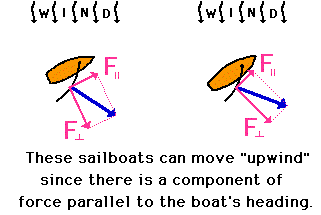The following problems focus on concepts discussed in this lesson. Answer each question and then click the button to view the answer.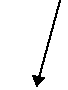1. The diagram at the right depicts a force that makes an angle to the horizontal. This force will have horizontal and vertical components. Which one of the choices below best depicts the direction of the horizontal and vertical components of this force?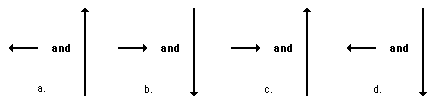2. Three sailboats are shown below. Each sailboat experiences the same amount of force, yet has different sail orientations.In which case (A, B or C) is the sailboat most likely to tip over sideways? Explain.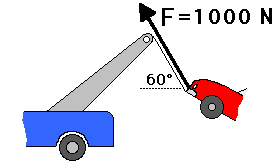3. Consider the tow truck at the right. If the tensional force in the cable is 1000 N and if the cable makes a 60-degree angle with the horizontal, then what is the vertical component of force that lifts the car off the ground?

Next Section: